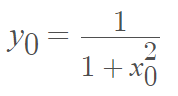# Witch of Agnesi

The Witch of Agnesi is a special case of a cubic hyperbola that is roughly bell-shaped.

The algebraic equation is

xy2 = a2(a x).

Alternatively, the curve can be turned 90° so that it lies on the horizontal axis:

yx2 = a2(a – y).

The parametric equations are :

• x(t) = at
• y(t) = a/(1 + t)2

The curve is named after the 18th century mathematician Maria Agnesi (1718 to 1799). It has a few surprising real life applications including as an approximation for the spectral line distribution of optical lines and x-rays. It also approximates the amount of power dissipated in resonant circuits .

## Finding Derivatives

The coordinates x0 and y0 satisfy the equationWe can take the derivative (with the chain rule) to get:## Construction of the Witch of Agnesi

The curve can be constructed as follows :

1. Choose a circle of diameter a.
2. Center the circle at (0, a/2)
3. Choose a point A on the line y = a; draw a line from A to the origin. Mark a new point B where this line crosses the circle.
4. If P is the point where the vertical line through P crosses the horizontal line through B, then the witch of Agnesi is the curve traced by P as A travels along the line y = a.

## Why is it Called the Witch of Agnesi?

It turns out, it’s named the “witch” because of a mistake in translation . Agnesi’s two-volume treatise on calculus included the curve, which she called versiera, which is Italian for “that-which-turns.” But when her work was translated into English by a Cambridge professor, he mistook the word for l’aversiera, which means “witch” in Italian.

## References

 Fifty Famous Curves, Lots of Calculus Questions, And a Few Answers.
 Applications to the Witch. Retrieved March 7, 2022 from: https://cs.appstate.edu/~sjg/wmm/final/agnesifinal/applications.pdf
 The Witch of Agnesi. Retrieved March 6, 2022 from: https://mathwomen.agnesscott.org/women/witch.htm
 Lienhard, J. No. 1741: THE WITCH OF AGNESI. Retrieved March 7, 2022 from: https://www.uh.edu/engines/epi1741.htm

CITE THIS AS:
Stephanie Glen. "Witch of Agnesi" From StatisticsHowTo.com: Elementary Statistics for the rest of us! https://www.statisticshowto.com/witch-of-agnesi/